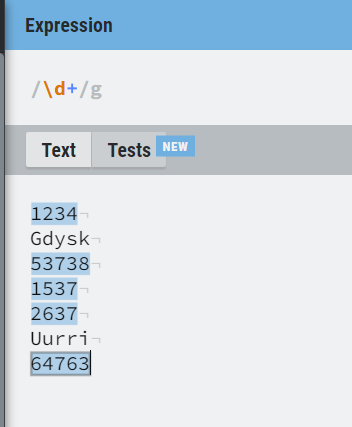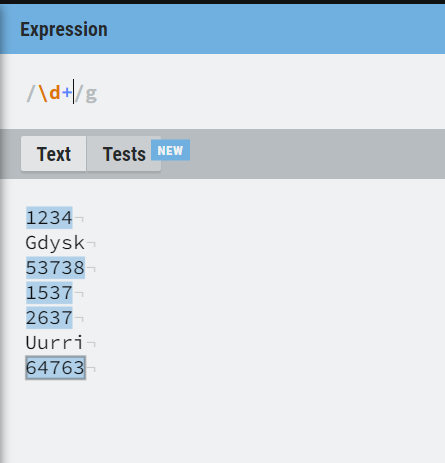# Take only number name file

Hi Team,
I have one folder in this folder multiple pdf files.
I want to get only name of pdf file only numbers.
Like
1234
Gdysk
53738
1537
2637
Uurri
64763

Try this

System.Text.RegularExpressions.Regex.IsMatch(yourstringinput.ToString,“(\d+)”)I hope it helps!!

try this

`\d+`

or esle assign the below syntax to a avriable

``````System.Text.RegularExpressions.Regex.Matches(sample.ToString,"\d+")
``````

in place of sample you can use your string variable which contains the data.

Regards

1 Like

Use the Regex expression to do thisStore all files in an list datatype variable

``````- Assign -> AllFiles = Directory.getfiles("Give your folder path here")
``````

After this
Use for each to loop to iterate the All files variable
Inside for each loop use If codition to check the file name contains numbers

In if condition give like this

``````System.Text.RegularExpressions.Regex.IsMatch(CurrentItem.GetFileNameWithOutExtension.ToString,“(\d+)”)
``````

Hope it helps!!

1 Like

If you want only then filenames which contain number then use as below

`Directory.GetFiles("Folderpath","*.pdf").Where(function(x) System.Text.RegularExpressions.Regex.IsMatch(Path.GetFileNameWithOutExtension(x),"\d+"))`

If you need only number related and not containing numbers then use

`Directory.GetFiles("Folderpath","*.pdf").Where(function(x) System.Text.RegularExpressions.Regex.IsMatch(Path.GetFileNameWithOutExtension(x),"^\d+\$"))`

This would give a array fo string which is array of filepaths

Cheers

1 Like

Try this:

``````(From file In Directory.GetFiles(folderPath, "*.pdf")
Let fileName = Path.GetFileNameWithoutExtension(file)
Let numbers = Regex.Matches(fileName, "\d+").Cast(Of Match)().Select(Function(m) m.Value)
From number In numbers
Select number).ToList()
``````
1 Like

Hi,
Assign: